Bitwise Operators are used to perform bit-level operations in C programming. They are also used for shifting of bits in the program.

There are six types of bitwise operators.

## 1. Bitwise AND operator `&`

In the Bitwise AND operation the output of the Bitwise AND operator will be 1 if both the bits are 1. If any of the two bits are 0 then the output will be 0.

For Example:

Consider A = 12 and B = 10 then Bitwise AND operation (A&B) will be

``````Binary of 12 is 00001100
Binary of 10 is 00001010

(A&B) will be

00001100
& 00001010
----------
00001000  = 8

Therefore (A&B) = 8
``````

The Bitwise AND Operation is based on the following truth table for two bits: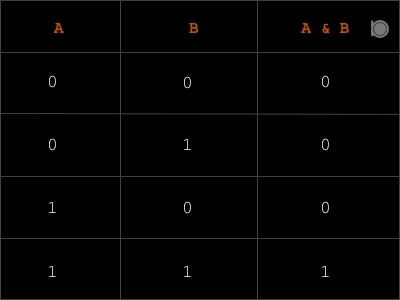### Program example of Bitwise AND Operator

``````//An example of Bitwise AND Operator
#include<stdio.h>

int main()
{
int A = 12, B = 10;
printf("Consider A = 12 and B = 10 \n");
printf("Bitwise AND Operation (A&B) = %d\n",(A&B));
return 0;
}
``````

### Output

``````Consider A = 12 and B = 10
Bitwise AND Operation (A&B) = 8
``````

## 2. Bitwise OR operator `|`

In the Bitwise OR operation the output of the Bitwise OR operator will be 1 if any of the bit is 1. If both of bits are 0 then the output will be 0.

For Example:

Consider A = 12 and B = 10 then Bitwise OR operation (A|B) will be

``````Binary of 12 is 00001100
Binary of 10 is 00001010

(A|B) will be

00001100
| 00001010
----------
00001110  = 14

Therefore (A|B) = 14
``````

The Bitwise OR Operation is based on the following truth table for two bits: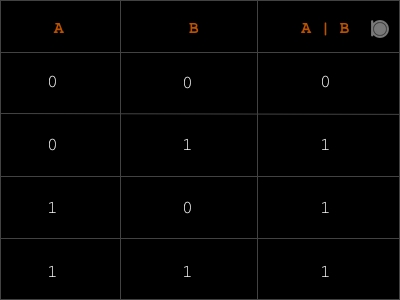### Program example of Bitwise OR Operator

``````//An example of Bitwise OR Operator
#include<stdio.h>

int main()
{
int A = 12, B = 10;
printf("Consider A = 12 and B = 10 \n");
printf("Bitwise OR Operation (A|B) = %d\n",(A|B));
return 0;
}
``````

### Output

``````Consider A = 12 and B = 10
Bitwise OR Operation (A|B) = 14
``````

## 3. Bitwise XOR operator `^`

In the Bitwise XOR operation the output of the Bitwise XOR operator will be 1 if both of the bits are opposite. If both of bits are same then the output will be 0.

For Example:

Consider A = 12 and B = 10 then Bitwise XOR operation (A^B) will be

``````Binary of 12 is 00001100
Binary of 10 is 00001010

(A^B) will be

00001100
^ 00001010
----------
00000110  = 6

Therefore (A^B) = 6
``````

The Bitwise XOR Operation is based on the following truth table for two bits: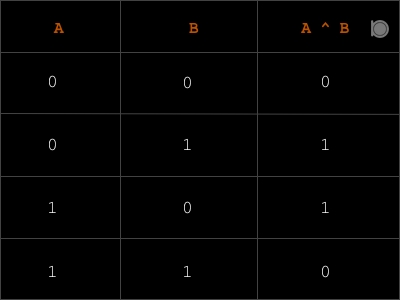### Program example of Bitwise XOR Operator

``````//An example of Bitwise XOR Operator
#include<stdio.h>

int main()
{
int A = 12, B = 10;
printf("Consider A = 12 and B = 10 \n");
printf("Bitwise XOR Operation (A^B) = %d\n",(A^B));
return 0;
}
``````

### Output

``````Consider A = 12 and B = 10
Bitwise XOR Operation (A^B) = 6
``````

## 4. Bitwise complement operator `~`

The Bitwise complement operator `~` is a unary operator. In the Bitwise complement operation the output of the Bitwise complement operator will be 1 if bit is 0 and output will be 0 if the bit is 1. But he Bitwise complement operation is abit twisted. It uses two’s complement system.

For Example:

Consider A = 12 then Bitwise Complement operation (~A) will be

``````Binary of 12 is 00001100

(~A) will be

~ 00001100
----------
11110011  = 243 in decimal but the computer
stores  integers  in  2's
complement form so
(11110011) would be -13

To understand this,
Look at the two's complement of 13 below

Binary of 13 is 00001101

One's complement of 13 is 11110010

Two's complement of 13 is 11110011 which is (-13)

Therefore (~A) = -13
``````

The Bitwise Complement Operation is based on the following truth table: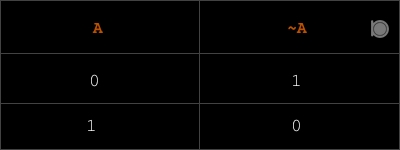### Program example of Bitwise Complement Operator

``````//An example of Bitwise Complement Operator
#include<stdio.h>

int main()
{
int A = 12;
printf("Consider A = 12 \n");
printf("Bitwise Complement Operation (~A) = %d\n",(~A));
return 0;
}
``````

## Output

``````Consider A = 12
Bitwise Complement Operation (~A) = -13
``````

## 5. Bitwise Shift Right `>>`

Bitwise Shift Right is used to shift the binary bits right by specific position.

For example:

Let a decimal number be 12.

And its binary is 00001100

Now if we shift bits of 12 right by 1 i.e. (12 » 1) then it would results 6.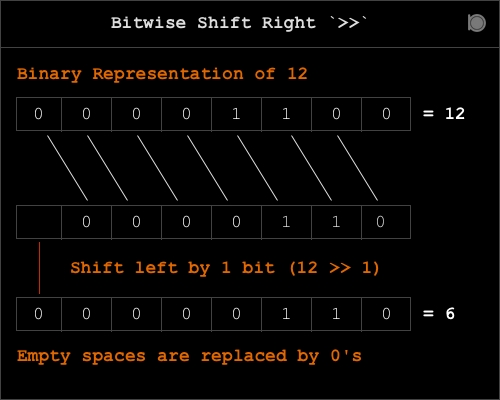Let’s take another example

Consider a decimal number be 36.

And its binary is 00100100.

Now if we shift bits of 36 right by 2 i.e (36 » 2) then it would results 9.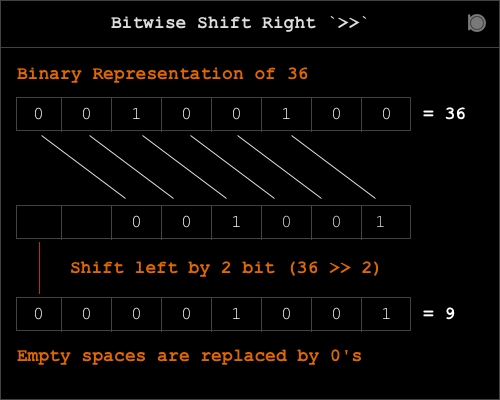This concludes that if `m` is the number and `n` is the bits to shift right the it follows the rule `m/2^n` .

### Program example of Bitwise Shift Right Operator

``````//An example of Bitwise Shift Right Operator
#include<stdio.h>

int main()
{
int A = 12, B = 36;
printf("Consider A = 12 and B = 36 \n");
printf("Bitwise Shift Right Operation (A >> 1) = %d\n",(A >> 1));
printf("Bitwise Shift Right Operation (B >> 2) = %d\n",(B >> 2));
return 0;
}
``````

### Output

``````Consider A = 12 and B = 36
Bitwise Shift Right Operation (A >> 1) = 6
Bitwise Shift Right Operation (B >> 2) = 9
``````

## 6. Bitwise Shift Left `<<`

Bitwise Shift Left is also similar to Bitwise Shift Right. It is used to shift the binary bits to Left by specific position.

For Example:

Let a decimal number be 12.

And its binary is 00001100.

Now if we shift bits of 12 left by 1 i.e. (12 « 1) then it would results 24.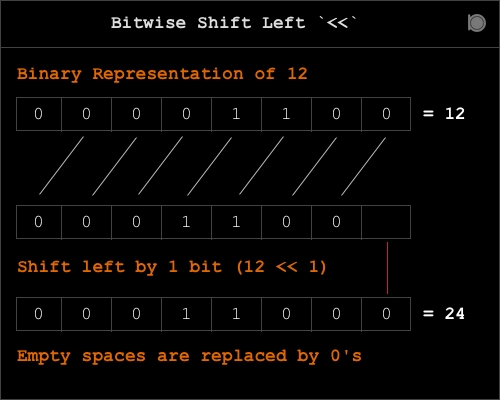Let’s take another example

Consider a decimal number be 2.

And its binary is 00000010.

Now if we shift bits of 2 left by 3 i.e (2 « 3) then it would results 16.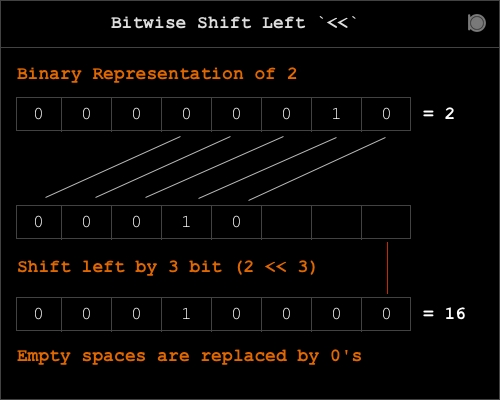This concludes that if `m` is the number and `n` is the bits to shift left the it follows the rule `m*2^n` .

### Program example of Bitwise Shift Left Operator

``````//An example of Bitwise Shift Left Operator
#include<stdio.h>

int main()
{
int A = 12, B = 2;
printf("Consider A = 12 and B = 2 \n");
printf("Bitwise Shift Left Operation (A << 1) = %d\n",(A << 1));
printf("Bitwise Shift Left Operation (B << 2) = %d\n",(B << 3));
return 0;
}
``````

### Output

``````Consider A = 12 and B = 2
Bitwise Shift Left Operation (A << 1) = 24
Bitwise Shift Left Operation (B << 2) = 16
``````